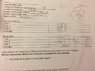# Catapult lab help needed please

alli66
[Note from mentor: This was originally posted in a non-homework forum, therefore it does not use the homework template.]

we did a lab on catapult but I'm having trouble figuring out the math? i calculated the hangtime, but all i did was take the original time and divide it by 2, is that right?
QUESTIONS:
1) how long did it take to reach peak height?
2) what is the projectiles initial vel in the x and y direction?
3) what was the initial vel.?
4) what was the peak height?
5) how much PE did the ball have at the peak height?use h from #4
6) what's the KE of the ball at the launch? use vi from #3
7) how fast is the ball moving at the peak height?use conservation of energy
8 )what was the elastic P.E of the catapult?
9) what was the spring constant?
10) how much work was done by gravity on the ball?

DATA:
- catapult launch angle 70 degrees
- length of armature = 28cm
- elastic band length before= .875in
- elastic band length after= 2.75 in
- mass of ball= 18.75 g

launch distance: trial 1: 12.85m, trial 2: 3.09m, trial 3: 3.15m, avg of the 3= 3.03 m
hang time: original time divided by 2 to get hang time? that's what i did but i think its wrong: trial 1: 1.60s/2 =.8 sec, trial 2: 1.65 sec/2= .825, trial 3: 1.71/2=.855s,, avg of 3 hangtimes= .8266secs

#### Attachments

•IMG_1195.jpg
57.2 KB · Views: 363
Last edited by a moderator: